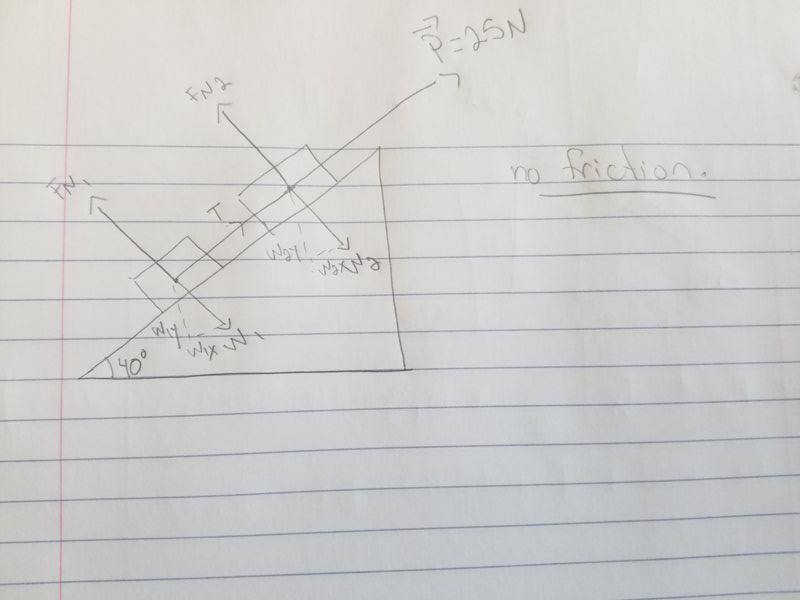# Using acceleration to find tension (concept problem)

Homework Statement:
Two boxes of masses M1= 2kg and M2 = 1 kg are pulled up a frictionless ramp by a force P=25N. Calculate the tension in the rope connecting the boxes. Theta is 40 degrees.
Relevant Equations:
F = m* a
Hi guys, I feel that this question has a very straightforward answer but I am just not quite grasping it. The first picture is the homework question, the second is the free body diagram I drew.

I know that a = F/m and I know the solution should be:

a = (P - m1*gsinθ - m2*gsinθ) / m1 + m2

What I don't understand is why isn't tension (T) included in the above equation? It's clearly along the x-axis with the others and P is used, so why not tension as well?

The equation that seems logical to me to use is:

a = (T + P - m1*gsingθ - m2*gsingθ ) / m1 + m2

but this is wrong. Can you help me understand why T doesn't belong?

#### Attachments

nasu
Gold Member
The tension acts on both objects not just on one of them. It is an internal force if you consider the system of the two objects.

•SkyOfMyOwnLight
Chestermiller
Mentor
If you we’re doing a force balance on each body individually, the tension T would come into each of the force balances. What would those two force balance equations be?

SammyS
Staff Emeritus
Homework Helper
Gold Member
Problem Statement: Two boxes of masses M1= 2kg and M2 = 1 kg are pulled up a frictionless ramp by a force P=25N. Calculate the tension in the rope connecting the boxes. Theta is 40 degrees.
Relevant Equations: F = m* a

Hi guys, I feel that this question has a very straightforward answer but I am just not quite grasping it. The first picture is the homework question, the second is the free body diagram I drew.

I know that a = F/m and I know the solution should be:

a = (P - m1*gsinθ - m2*gsinθ) / m1 + m2

What I don't understand is why isn't tension (T) included in the above equation? It's clearly along the x-axis with the others and P is used, so why not tension as well?

The equation that seems logical to me to use is:

a = (T + P - m1*gsingθ - m2*gsingθ ) / m1 + m2

but this is wrong. Can you help me understand why T doesn't belong?

Hello, @SkyOfMyOwnLight .The given solution treats the two boxes along with the connecting rope as one object.

By the way: the sum of the masses, m1 + m2, should be enclosed in parentheses if you mean for it all to be in the denominator as in the following.

a = (P - m1⋅g⋅sinθ - m2⋅g⋅sinθ) / (m1 + m2)

This acceleration can then be used to determine the tension, T .